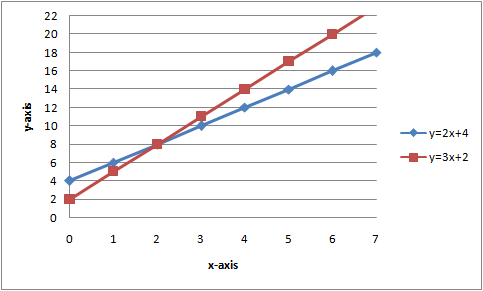# Graphing linear systems

A system of linear equation comprises two or more linear equations. The solution of a linear system is the ordered pair that is a solution to all equations in the system.

One way of solving a linear system is by graphing. The solution to the system will then be in the point in which the two equations intersect.

Example

Solve the following system of linear equations

$\left\{\begin{matrix} y=2x+4\\ y=3x+2 \end{matrix}\right.$The two lines appear to intersect in (2, 8)

It's a good idea to always check your graphical solution algebraically by substituting x and y in your equations with the ordered pair

$\underline{y=2x+4} \\ {\color{green} 8}\overset{?}{=} 2\cdot {\color{green} 2}+4$

$8=8$

$\underline{y=3x+2}$

${\color{green} 8}\overset{?}{=}3\cdot {\color{green} 2}+2$

$8=8$

A linear system that has exactly one solution is called a consistent independent system. Consistent means that the lines intersect and independent means that the lines are distinct.

Linear systems composes of parallel lines that have the same slope but different y-intersect do not have a solution since the lines won't intersect. Linear systems without a solution are called inconsistent systems.

Linear systems composed of lines that have the same slope and the y-intercept are said to be consistent dependent systems. Consistent dependent systems have infinitely many solutions since the lines coincide.

## Video lesson

Solve the linear system graphically

$\left\{\begin{matrix} 2y - 4x = 2 \\ y = -x + 4\\ \end{matrix}\right.$